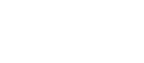Phil Bergeron-Burns

# C++ Simulation Code for MIMO Signals

## Introduction

I wrote this code quickly to justify the research I was doing for my master's thesis in 2002-2003. It's mostly written in C but there are some small elements that used C++ features. The compiled code runs quite quickly compared to similar code running in an interpreted environment, such as Matlab: I was able to generate error probabilities valid up to 10-5 usually overnight, while my lab colleagues sometimes took over a week.

A tarball of the code (everything you need to run the simulations) can be downloaded as open source. The code is also available at github. I welcome any suggestions, fixes, and comments.

To better understand the context and assumptions made for these simulations, see my work. I can provide a brief summary of the assumptions if anyone is interested.

```
This README file explains how to use the C++ programs that were used
to generate the simulations in this thesis.  The capabilities and
limitations of these programs will also be discussed.  An overview
of the program source code files which appear later in this
appendix can be found in the following table.

Source file name     Description
================     ===========

uncodedMIMO.cpp      The uncoded simulation entry point and primary flow.
LDcodedMIMO.cpp      The LD coded simulation entry point and primary flow.
sphere1.cpp          Implements the SD based on the flowchart of .
sphere2.cpp          Implements the SE/SD based on the flowchart of .
QR.cpp               Does the QR factorization on an arbitrary matrix.
utilities.cpp        Miscellaneous procedures used in the simulation.
matrix.cpp           Implements some required matrix operations.

To run a simulation put all of the above files in the same
directory. These programs have been successfully compiled and run
using the Linux standard g++ version 3.2.3-42 compiler and the
Microsoft Visual C++ version 6.0 compiler.  To run the simulation
of the uncoded framework compile uncodedMIMO.cpp and run the
executable and redirect the standard output to a text file.  For
example, you can simulate the uncoded framework on the Linux
operating system by running the following commands:

Linux> g++ uncodedMIMO.cpp -o execute_test
Linux> execute_test > output_test_file.txt

When the execute_test executable finishes the text file
output_test_file.txt stores the results.  To run the simulation
of the LD coded framework compile the LDcodedMIMO.cpp file.  For
example, you can simulate the coded framework on the Linux
operating system by running the following commands:

Linux> g++ LDcodedMIMO.cpp -o execute_coded
Linux> execute_coded > output_coded_file.txt &

The uncoded MIMO simulation has the following constants that are

Description                               Allowable values
===========                               ================

The number of transmit antennas.          1 <= ACTUAL_NUM_TX <= 25
The number of receive antennas.           1 <= ACTUAL_NUM_RX <= 25
The size of the symbol constellation.     PAM = {2,4,8,16}
The minimum number trials per SNR.        1 <= TRIALS
The minimum number of
symbol errors per SNR.                    0 <= MIN_ERROR
The SPC front-end U parameter.            0 <= SPC_NOISE
The SFC front-end T parameter.            0 <= pure_Ph
Channel matrix elements error variance    0 <= VAR_UNCERT

The SNR values for which can also be set in the uncoded simulation
and in the LD coded simulation.  The adjustable variables are the
same in the LD coded simulation except that the number of transmit